11. Input devices¶

Assignment¶

• Group assignment
Measure the analog levels and digital signals in an input device

• Individual assignment
Measure something: add a sensor to a microcontroller board that you have designed and read it.

Planning¶

My plan is to use light (visible) sensor for my board. It will be used for my final project to detect user interaction. For example, a user put a wood branch into a hole of the table I created at week8, then the output of the mist changes accordingly.Light sensors (visible) PT15-21C/TR8 / Data sheet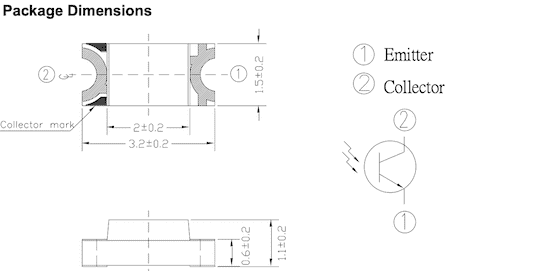Board example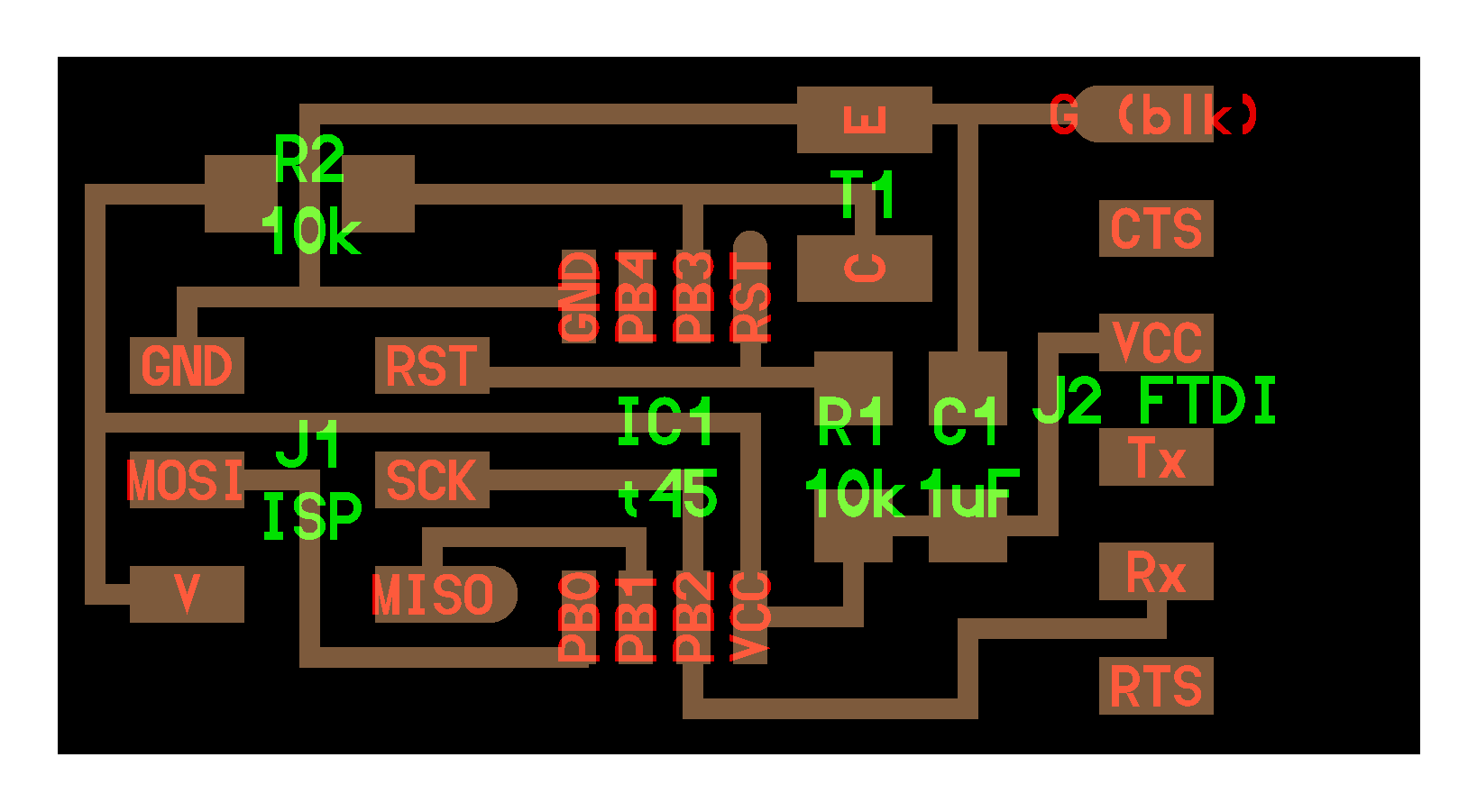Based on the example board, I modified following points to fit the final project plan.
I first planned to use five ADC ports of an ATTINT44 for sensors. But my instructor advised me that if you might change the number, it might be interesting to divide sensors into each micon board and network them. So I decided to try the scalable plan.

• ATTINY45 –> ATTINY44
• Extra serial port to network with other sensor boards.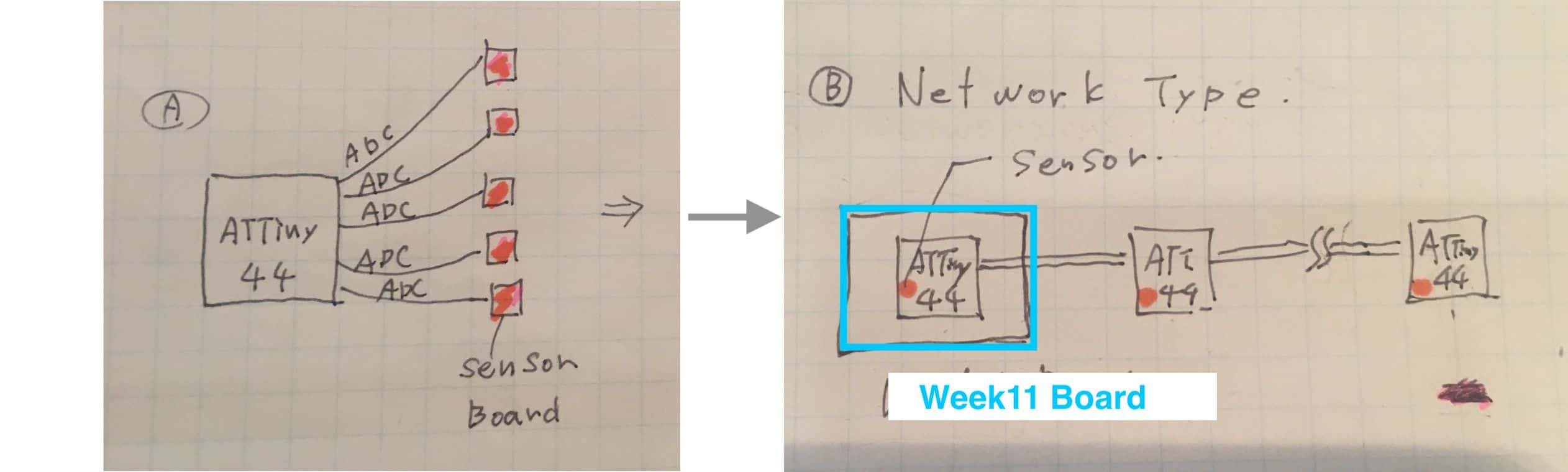Parts List

parts description number
micro controller ATTiny 44 1
Light sensor PT15-21C/TR8 1
capacitor 1uF 1
resistor 10KΩ 2
resistor 0KΩ 2

Design¶

I designed the board with Eagle.To make the schematics was not difficult as I referred the example, however the routing was very tough work for me. I first laid out the parts the following left pattern, but I couldn’t connect properly. So I tried right pattern and struggled for hours, then finally I did it with two jumpers (0Ω resister).At DRC, the two jumper points were listed up as Airwire errors as follows. So I approved them.The long name was affected the export of png image.　Unnecessary space was created in the png file, so I shortened the name.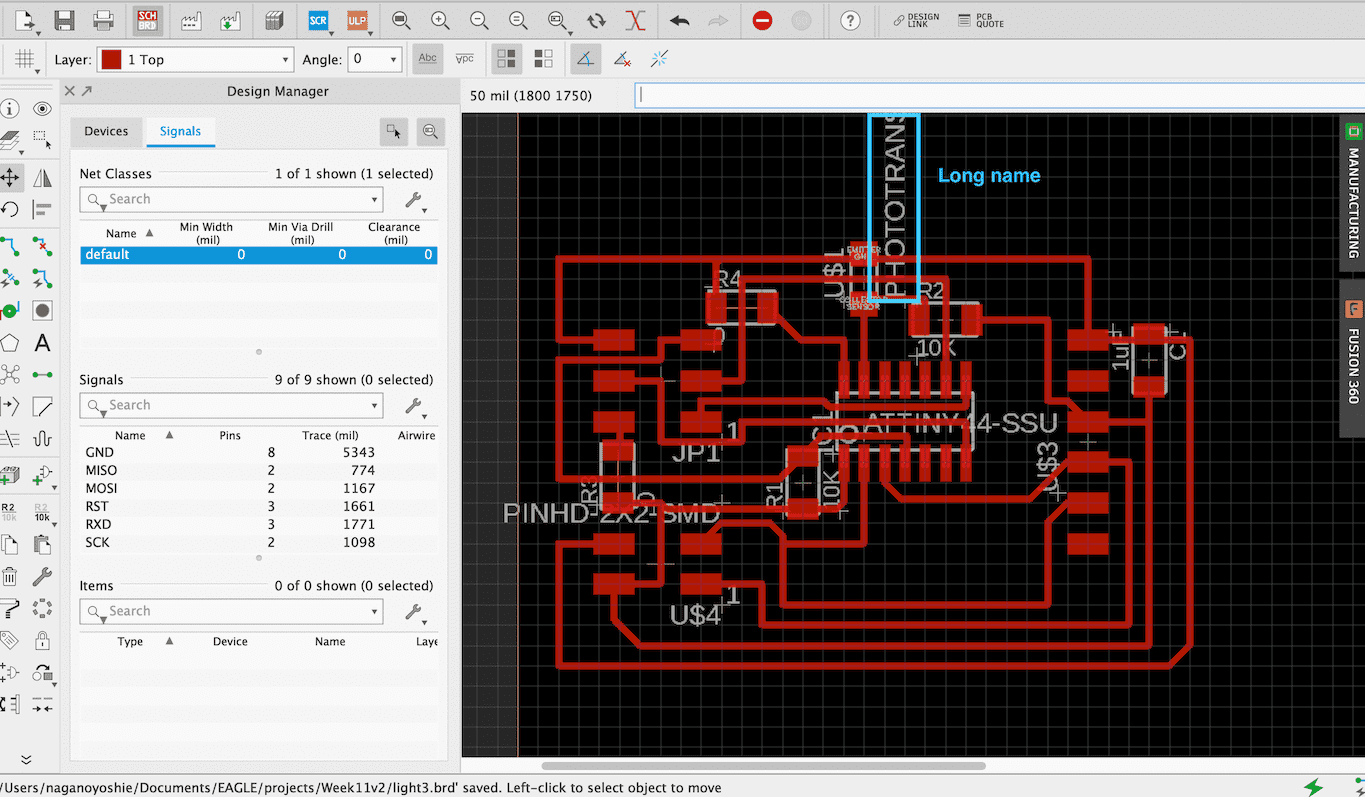The designed board ant outline are as follows.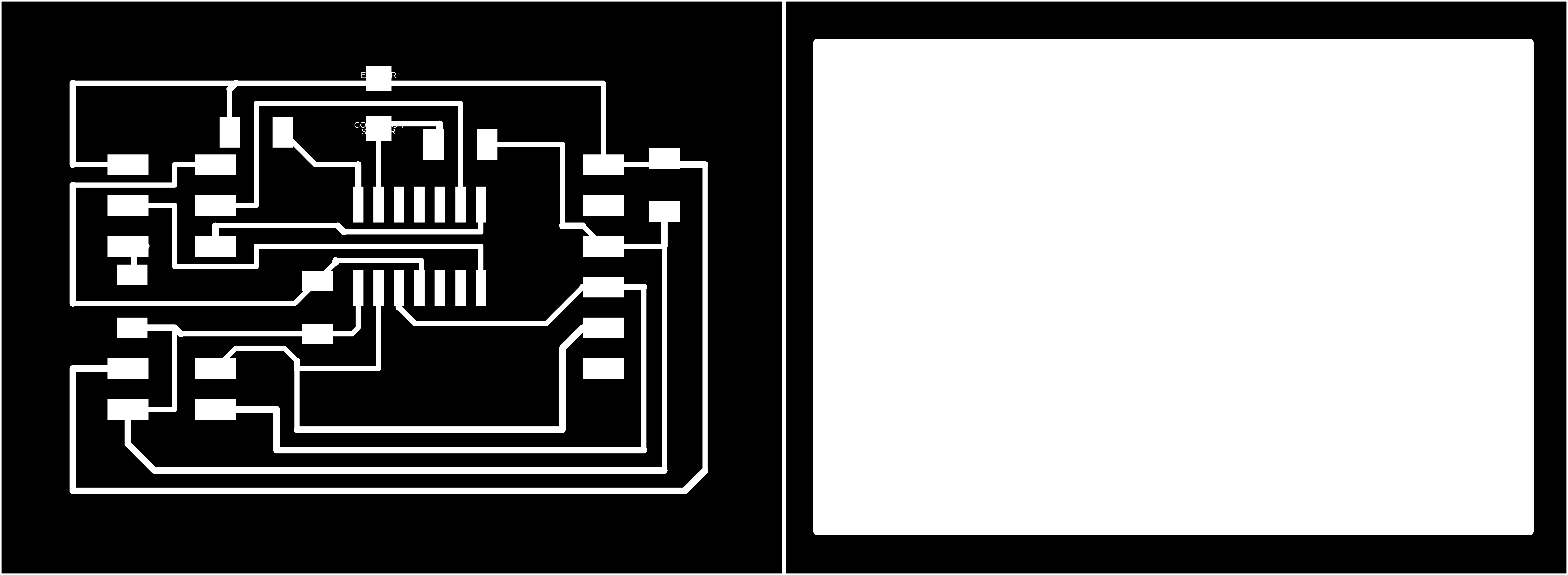Milling¶

I milled the board using SRM-20 and soldered the parts.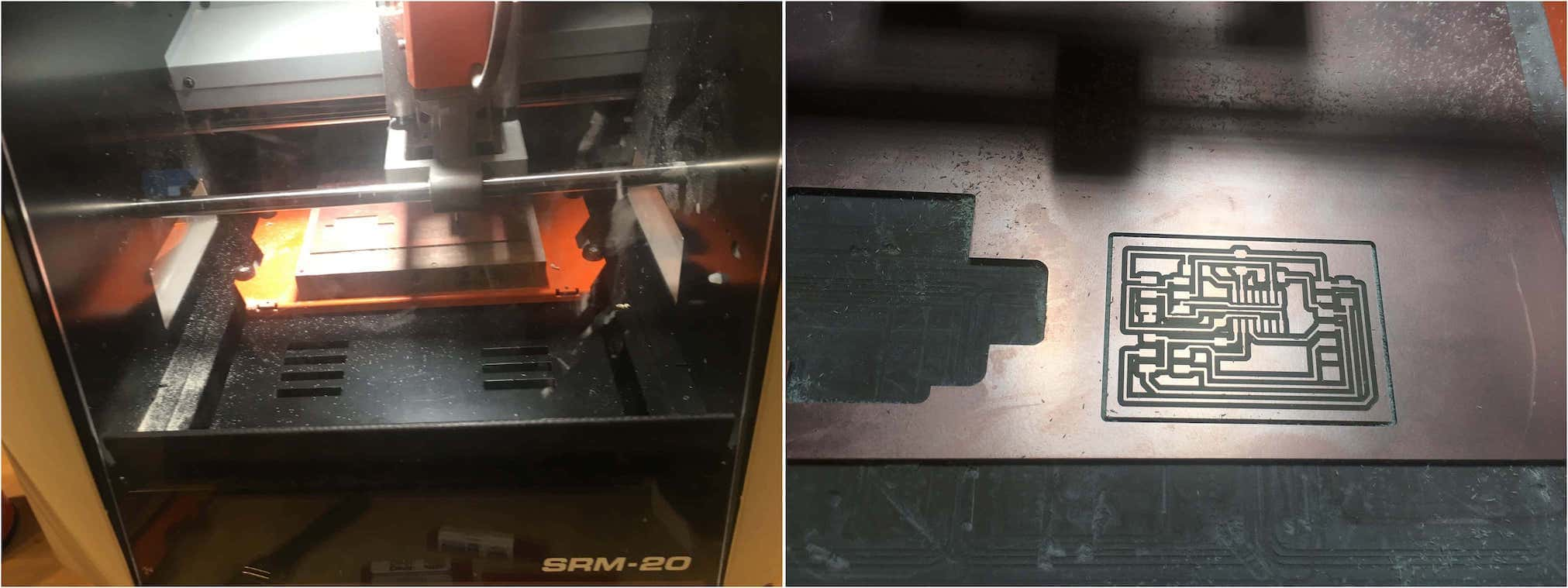I realized that the capacitor between VCC & GND would prevent the soldering of header. So I relocated the capacitor to beside the header. It is important to design the board considering the 3d shape of each parts.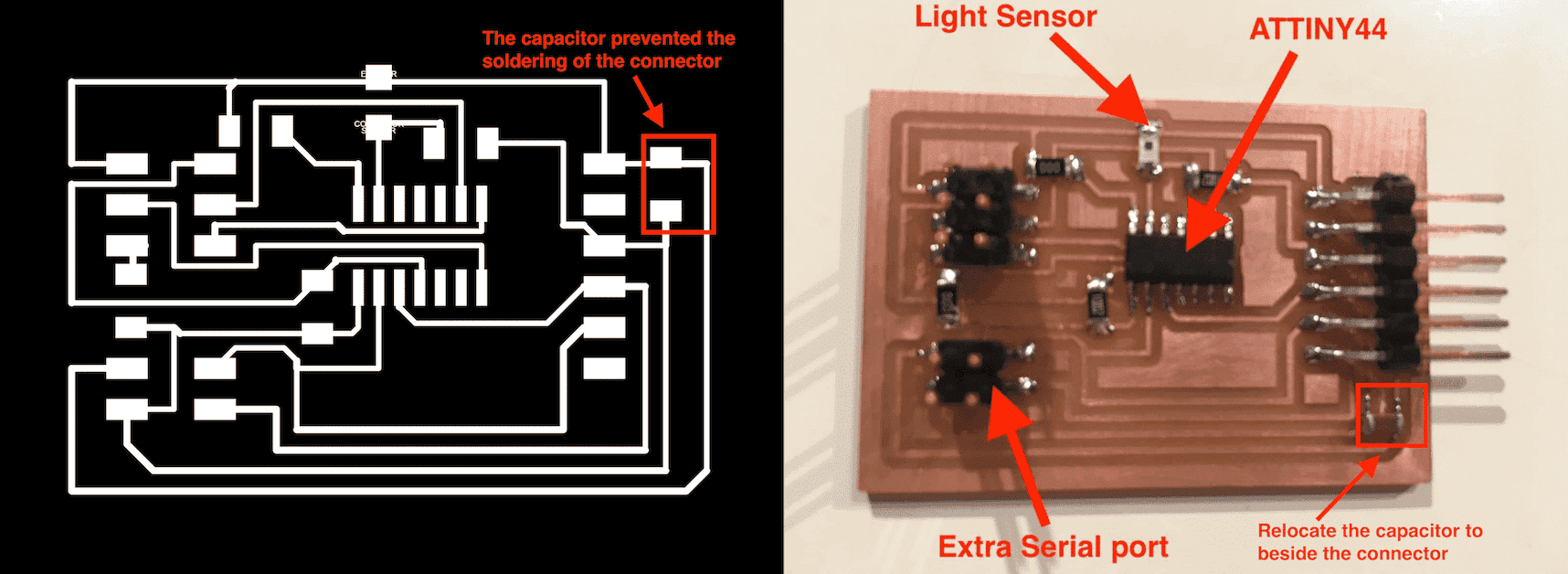First I couldn’t measured the analogue signal with oscilloscope. I realized that I soldered the light sensor at opposite orientation. So I changed the orientation, then I could measure the signal as follows.

C programming¶

I modified the example code of ATTINY45 to ATTINY44. Some of the registers are different from ATTINY45, so I read the ATtiny44A datasheet carefully.

Changes from ATtiny45 to ATtiny44
- MUX : Add MUX4 and MUX5

ADMUX is the ADC Multiplexer Selection Register. Initialization value is as follows.
- VCC used as analog reference (REFS1,0 = 00)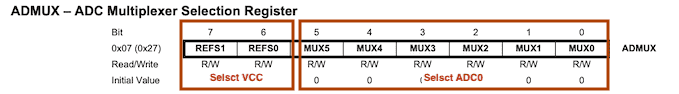ADMUX = (0 << REFS1) | (0 << REFS0) // Vcc ref
| (0 << MUX5) | (0 << MUX4) | (0 << MUX3) | (0 << MUX2) | (0 << MUX1) | (0 << MUX0); // ADC0

- Prescaler = 128 (ADPS2,1,0 = 111)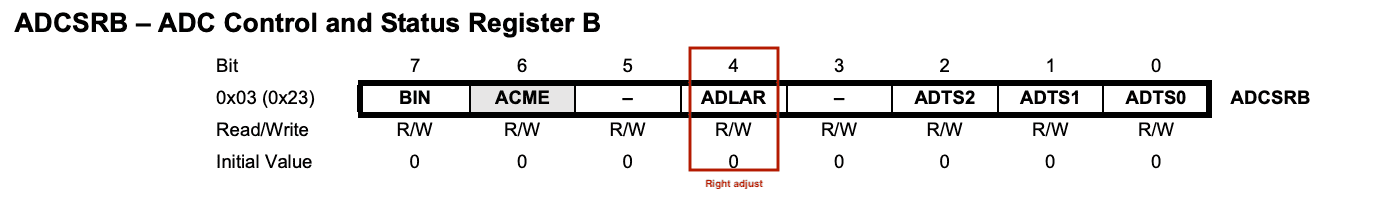ADC timing chart is as follows. When the ADSC bit of ADSCRA is set, the conversion starts. A normal conversion takes 13 ADC clock cycles, however takes 25 clock in case of first conversion after the ADEN bit is set.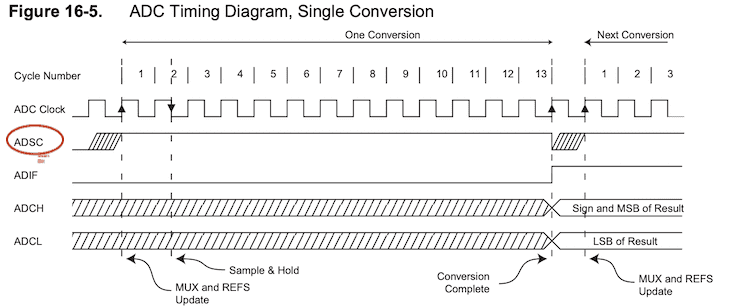After the conversion is complete (ADSC is Low), the conversion result can be found in the ADC Data Registers (ADCL, ADCH). As the data is 10 bit, combine the High part(ADCL) and Low part(ADCH) as follows.

// initiate conversion
//
//
// wait for completion
//
;
//
// send result
//
put_char(&serial_port, serial_pin_out, chr);
char_delay();
put_char(&serial_port, serial_pin_out, chr);

Test serial data¶

I tested the serial data using Arduino serial monitor. The framing code “1234” are not ASCII, so I changed them to visible alphabet code ‘F’ ‘A’ ‘B’ ‘K’ temporary to check the serial communication. The alphabet codes were displayed properly as follows, so I confirmed the serial function.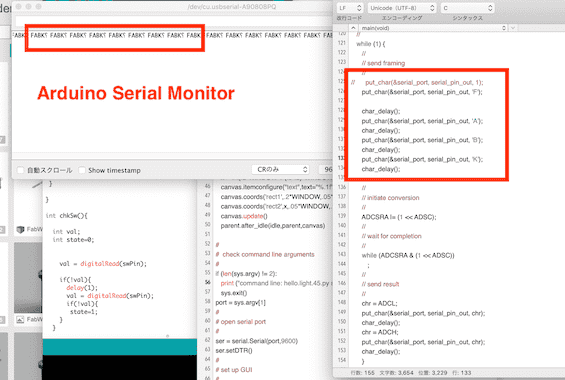C code¶

//======================================================
//        light.44.c
//======================================================
#include <avr/io.h>
#include <util/delay.h>

#define output(directions,pin) (directions |= pin) // set port direction for output
#define set(port,pin) (port |= pin) // set port pin
#define clear(port,pin) (port &= (~pin)) // clear port pin
#define pin_test(pins,pin) (pins & pin) // test for port pin
#define bit_test(byte,bit) (byte & (1 << bit)) // test for bit set
#define bit_delay_time 102 // bit delay for 9600 with overhead
#define bit_delay() _delay_us(bit_delay_time) // RS232 bit delay
#define half_bit_delay() _delay_us(bit_delay_time/2) // RS232 half bit delay
#define char_delay() _delay_ms(10) // char delay

#define serial_port PORTB
#define serial_direction DDRB
#define serial_pin_out (1 << PB0)   //week11

void put_char(volatile unsigned char *port, unsigned char pin, char txchar) {
//
// send character in txchar on port pin
//    assumes line driver (inverts bits)
//
// start bit
//
clear(*port,pin);
bit_delay();
//
// unrolled loop to write data bits
//
if bit_test(txchar,0)
set(*port,pin);
else
clear(*port,pin);
bit_delay();
if bit_test(txchar,1)
set(*port,pin);
else
clear(*port,pin);
bit_delay();
if bit_test(txchar,2)
set(*port,pin);
else
clear(*port,pin);
bit_delay();
if bit_test(txchar,3)
set(*port,pin);
else
clear(*port,pin);
bit_delay();
if bit_test(txchar,4)
set(*port,pin);
else
clear(*port,pin);
bit_delay();
if bit_test(txchar,5)
set(*port,pin);
else
clear(*port,pin);
bit_delay();
if bit_test(txchar,6)
set(*port,pin);
else
clear(*port,pin);
bit_delay();
if bit_test(txchar,7)
set(*port,pin);
else
clear(*port,pin);
bit_delay();
//
// stop bit
//
set(*port,pin);
bit_delay();
//
// char delay
//
bit_delay();
}

int main(void) {
//
// main
//
static char chr;
//
// set clock divider to /1
//
CLKPR = (1 << CLKPCE);
CLKPR = (0 << CLKPS3) | (0 << CLKPS2) | (0 << CLKPS1) | (0 << CLKPS0);
//
// initialize output pins
//
set(serial_port, serial_pin_out);
output(serial_direction, serial_pin_out);
//
// init A/D
//
// ADMUX = (0 << REFS2) | (0 << REFS1) | (0 << REFS0) // Vcc ref
ADMUX = (0 << REFS1) | (0 << REFS0) // Vcc ref week11
//  | (0 << MUX3) | (0 << MUX2) | (1 << MUX1) | (1 << MUX0); // ADC3
| (0 << MUX5) | (0 << MUX4) | (0 << MUX3) | (0 << MUX2) | (0 << MUX1) | (0 << MUX0); // ADC0 week11

//
// main loop
//
while (1) {
//
// send framing
//
put_char(&serial_port, serial_pin_out, 1);
//     put_char(&serial_port, serial_pin_out, 'F');

char_delay();
put_char(&serial_port, serial_pin_out, 2);
char_delay();
put_char(&serial_port, serial_pin_out, 3);
char_delay();
put_char(&serial_port, serial_pin_out, 4);
char_delay();

//
// initiate conversion
//
//
// wait for completion
//
;
//
// send result
//
put_char(&serial_port, serial_pin_out, chr);
char_delay();
put_char(&serial_port, serial_pin_out, chr);
}
}

Application : Processing¶

I programmed processing to visualize the light sensor signal. The upper one is real time graph, and lower one shows the change of time axis.
I confirmed that the analogue signal changed properly according to the hand interaction.

Processing code:

/****************************************************************************
Week11 Input device
Light sensor grough
*****************************************************************************/

import processing.serial.*;

Serial myPort;  // Create object from Serial class
int val;      // Data received from the serial port
int cnt= 0;
int ltL=0;
int ltH=0;
int ltDat=0;
int xx=0;

float eps = 0.5;
float filter = 0.0;

void setup()
{
size(550,300);
myPort = new Serial(this, "/dev/cu.usbserial-A90808PQ", 9600);
background(255);             // Set background to white
}

void draw()
{

noStroke();
fill(0, 130, 130);
text("Light Sensor ", 50,25);

float x1= map ( filter, 0, 1024, 0, 450);
fill(172, 255, 255);
rect(50, 50, 450, 50);
fill(0, 130, 130);
rect(50, 50, x1, 50);

float x2 = map ( filter, 0, 1024, 0, 100);
fill(0,130,130);
rect(50+xx, 120, 1, 100);
fill(172, 255, 255);
rect(50+xx, 120, 1, 100-x2);

if(xx++ == 450){xx=0;};

}

void serialEvent(Serial myPort) {
}

// println(val);
// println ("cnt = " + cnt);

switch(cnt){
case 0:
if(val==1){ cnt = 1;}
break;
case 1:
if(val==2){ cnt = 2;
}else if(val!=1) {
cnt=0;
}
break;
case 2:
if(val==3){ cnt = 3;
}else{
cnt=0;
}
break;
case 3:
if(val==4){ cnt = 4;
}else{
cnt=0;
}
break;
case 4:
ltL = val;
cnt = 5;
break;
case 5:
ltH = val;
ltDat = 256*ltH + ltL;
filter = (1-eps)*filter + eps*ltDat;
println ("filter = " + filter);
cnt = 0;

break;
}

}